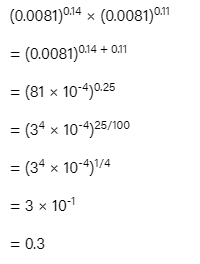# Quantitative Aptitude Quiz for UPSI: 14 April 2021

Updated Wed, 14 Apr 2021 08:00 PM ISTSource: safalta
1. Simplify {(9) - (-8) + (24 ÷ 13 - 7)}

a) 154/13
b) 155/13
c) 157/13
d) 158/13

Given expression is {(9) - (-8) + (24 ÷ 13 - 7)}
⇒ 17 + (24/13) - 7
⇒ 10 + 24/13
⇒ 154/13

2. Simplify: (0.0081)^0.14 × (0.0081)^0.11

a) 0.1
b) 0.3
c) 0.6
d) 0.9### Free Demo Classes

3. What will come in place of question Mark ‘?’ in the following number question?
(756 + 62 × ?) ÷ 3 = 57 × 9 + 49

a) 12
b) 13
c) 14
d) 15

⇒ (756 + 62 × ?) ÷ 3 = 57 × 9 + 49

⇒ (756 + 62 × ?) ÷ 3 = 513 + 49

⇒ (756 + 62 × ?) ÷ 3 = 562

⇒ (756 + 62 × ?) = 562 × 3

⇒ (756 + 62 × ?) = 1686

⇒ 62 × ? = 1686 - 756

⇒ 62 × ? = 930

⇒ ? = 930 / 62

⇒ ? = 15

4. What will come in place of question mark in the following question?
0.000033 ÷ 0.11 = ?

a) 0.000003
b) 0.0003
c) 0.00003
d) 0.003

0.000033 ÷ 0.11 = ?

⇒ ? = 33/1000000 × 100/11

⇒ ? = 0.0003

5. A student’s mark was wrongly entered as 108 instead of 98. Due to this mistake, the average marks of the class got increased by 1/5. Total number of students in the class is equal to

a) 40
b) 45
c) 50
d) 60

Let, number of students in class = x

Average marks of the class = y

According to the question,

⇒ x(y + 1/5) = xy + 108 – 98

⇒ xy + x/5 = xy + 10

⇒ x/5 = 10

⇒ x = 50

∴ Number of students in the class = 50

6. The average of 11 observations is 60. If the average of first six observations is 59 and that of last six observations is 62, then the value of sixth observation, is:

a) 63
b) 64
c) 65
d) 66

Sum of 11 observation = 60 × 11 = 660
Sum of first 6 observation = 6 × 59 = 354
Sum of last 6 observation = 6 × 62 = 372
354 + 372 = 726
726 – 660 = 6th Observation = 66
∴ 6th observation is 66.

7. Average of 30 numbers is 55. If a number 70 replaced by 85, then the new average will be?

a) 58.5
b) 57.5
c) 56.5
d) 55.5

Sum of 30 numbers = 55 × 30 = 1650

New sum of 30 numbers = 1650 – 70 + 85 = 1665

∴ New average of 30 numbers = 1665/30 = 55.5

8. A batsman scores 91 runs in his 19th innings. Due to this his average increased by 4 runs. Find his current average.

a) 18
b) 19
c) 20
d) 21

Let the old average of batsman be x, then current average = x + 4

Now, x × 18 + 91 = (x + 4) × 19

⇒ 18x + 91 = 19x + 76

⇒ x = 91 - 76 = 15

∴ Current average = x + 4 = 15 + 4 = 19

9. If the length of each side of a square is increased by 15%, then the increase percent in its area is:

a) 32.25%
b) 33.25%
c) 34.25%
d) 35.25%

let each side of the square be a, then area = a^2

As given that the side is increased by 15%, then

New side = 115a/100 = 23a/20

New area = (23a/20)^2

Increased area = (529a^2/400) – a^2

Increase area% = [129a^2/400]/a^2 × 100 = 32.25%

10. If the income of A is 20% less than income of B, then how much income of B is more than income of A?

a) 20%
b) 25%
c) 30%
d) 15%

Let the income of B be 100

⇒ The income of A will be 20% less than A i.e. 80

∴ The income of B is more than A by 20

∴ Percentage of income of B is more than A by [(100 - 80)/80] × 100 = 25%

### Disclaimer

अपनी वेबसाइट पर हम डाटा संग्रह टूल्स, जैसे की कुकीज के माध्यम से आपकी जानकारी एकत्र करते हैं ताकि आपको बेहतर अनुभव प्रदान कर सकें, वेबसाइट के ट्रैफिक का विश्लेषण कर सकें, कॉन्टेंट व्यक्तिगत तरीके से पेश कर सकें और हमारे पार्टनर्स, जैसे की Google, और सोशल मीडिया साइट्स, जैसे की Facebook, के साथ लक्षित विज्ञापन पेश करने के लिए उपयोग कर सकें। साथ ही, अगर आप साइन-अप करते हैं, तो हम आपका ईमेल पता, फोन नंबर और अन्य विवरण पूरी तरह सुरक्षित तरीके से स्टोर करते हैं। आप कुकीज नीति पृष्ठ से अपनी कुकीज हटा सकते है और रजिस्टर्ड यूजर अपने प्रोफाइल पेज से अपना व्यक्तिगत डाटा हटा या एक्सपोर्ट कर सकते हैं। हमारी Cookies Policy, Privacy Policy और Terms & Conditions के बारे में पढ़ें और अपनी सहमति देने के लिए Agree पर क्लिक करें।

Agree

Get free counselling session